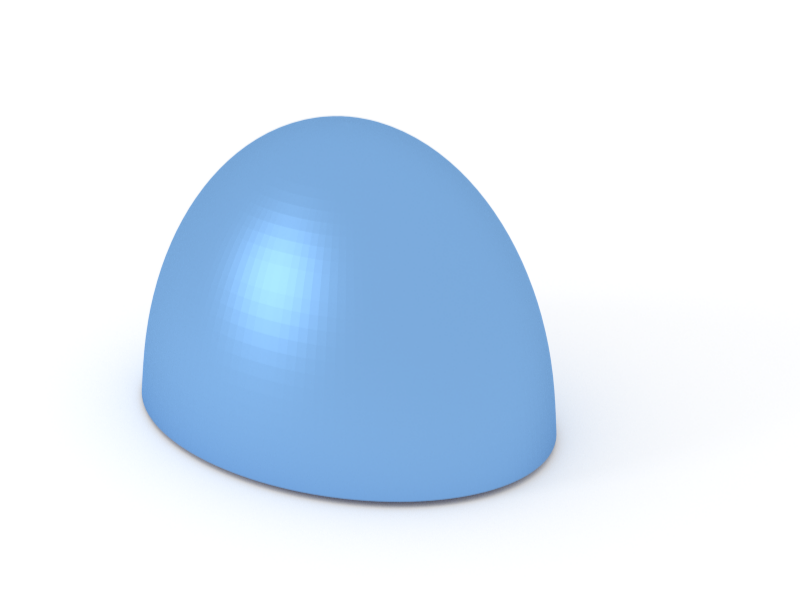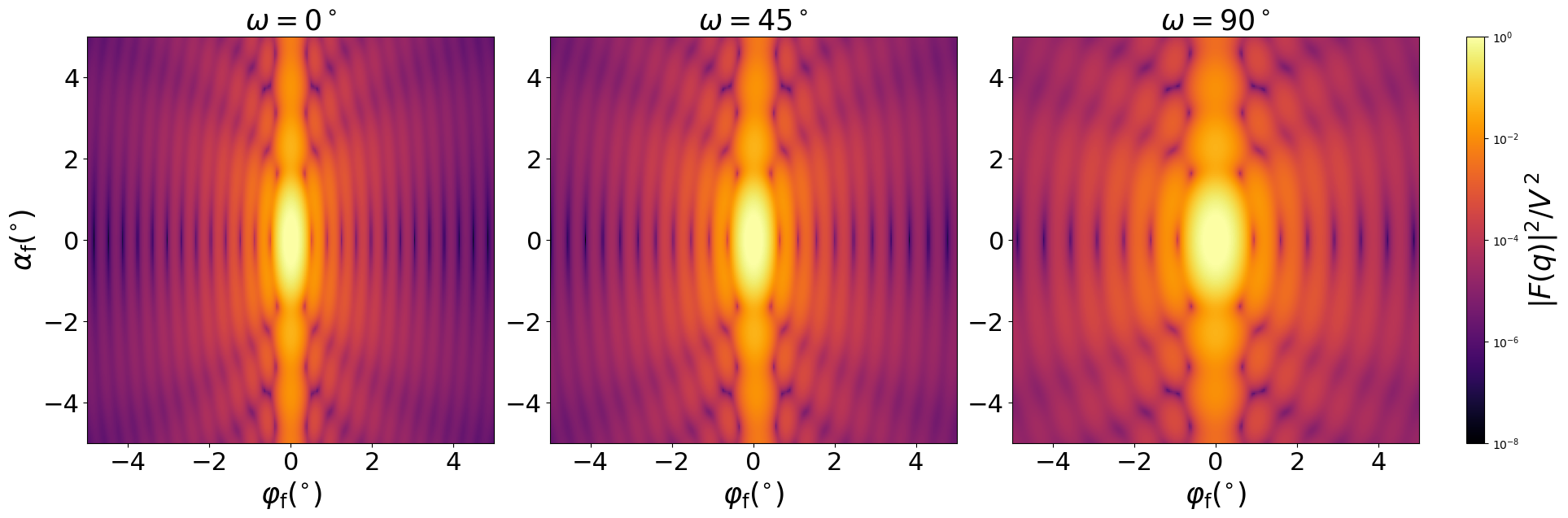### HemiEllipsoid

An horizontally oriented ellipsoid, truncated at the central plane.#### Constructor

HemiEllipsoid(R_a, R_b, H)


Parameters:

• R_a, radius $R_{a}$ in $x$ direction
• R_b, radius $R_{b}$ in $y$ direction
• H, height

#### Usage

As for any other Form factor.

#### Implementation

Class HemiEllipsoid inherits from the interface class IFormFactor .

Computation involves numerical integration in vertical direction,

$$F(\mathbf{q})=2\pi \int_{0}^H \text{d}z \space r_{a,z} r_{b,z} \frac{J_1(\gamma_{z})}{\gamma_{z}} \thinspace \exp(iq_z z) ,$$

with the notation

$$r_{a,z}:=R_{a}\sqrt{1-\Big( \dfrac{z}{H} \Big)^2}, \quad r_{b,z}:=R_{b}\sqrt{1-\Big( \dfrac{z}{H} \Big)^2},$$

$$\quad \gamma_{z} := \sqrt{(q_xr_{a,z})^2 + (q_yr_{b,z})^2}.$$

Volume has been validated against $$V=\dfrac{2\pi}{3} R_aR_bH.$$

#### Example

Scattering by uncorrelated, oriented hemiellipsoids for horizontal incidence. Rotation around $z$ axis:Generated by Examples/ff/HemiEllipsoid.py .

#### History

Agrees with the IsGISAXS form factor “Anisotropic hemi-ellipsoid” [manual, Eq. 2.41] with wrong sign in the z-dependent phase factor and “Hemi-spheroid” [Renaud 2009, Eq. 229].